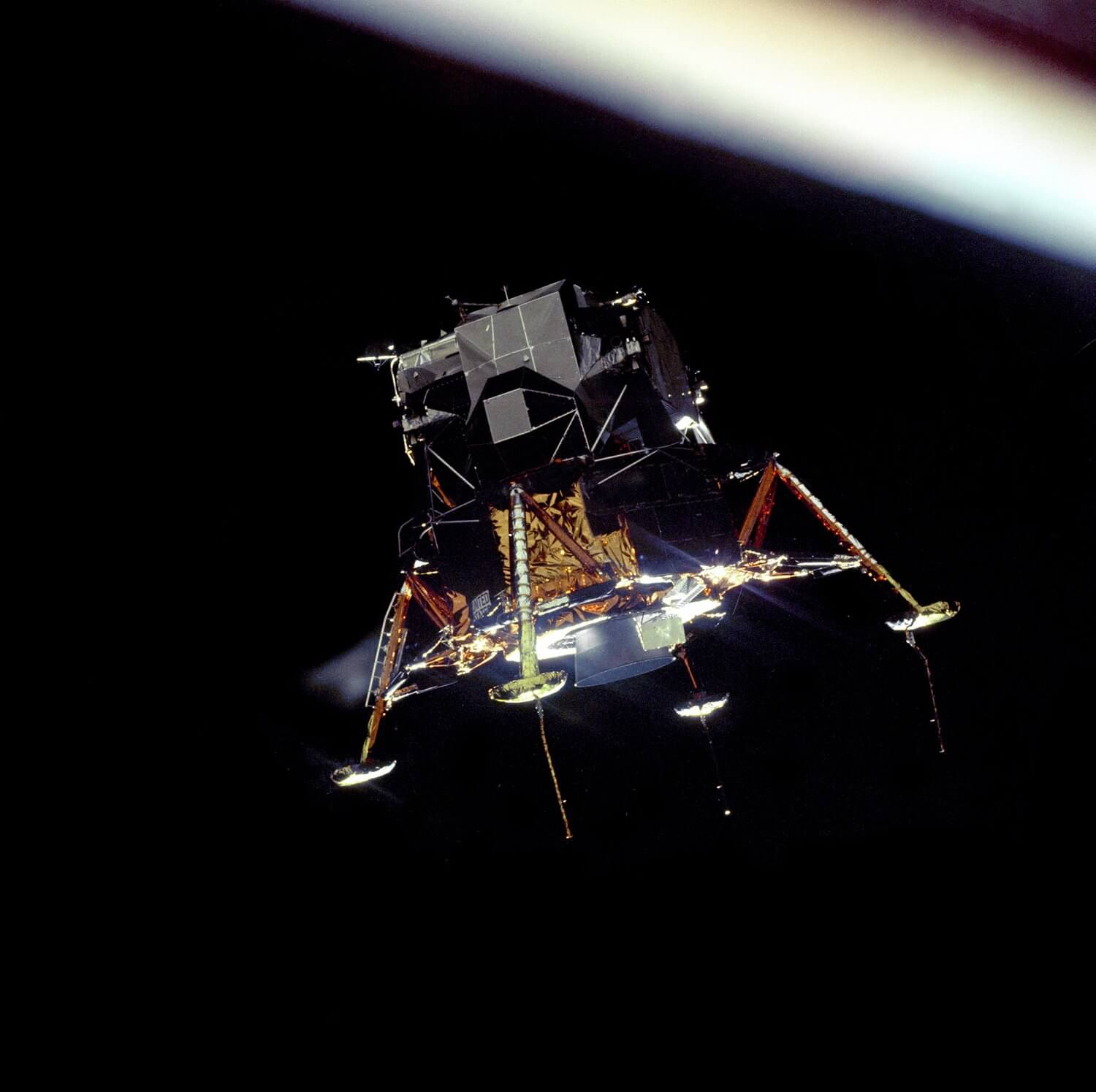🍎

# Physics

Tags Classical Mechanics @May 13, 2020 12:19 PM @July 11, 2021 7:17 PM

# Units

Billon is $10^9$﻿ in English, Portuguese, and Greek -"Mil millones" in Spanish. But, It's $10^{12}$﻿ in Spanish -trillion in English.

# Motion

1. $\quad v=v_0+at \quad$﻿, falta el desplazamiento ($\Delta x$﻿)

2. $\quad {\Delta x}=(\dfrac{v+v_0}{2})t$﻿, falta la aceleracion $(a)$﻿

3. $Δx=v_0​t+\dfrac{1}{2}at^2$﻿, falta v

4. $\quad v^2=v_0^2+2a$﻿, falta t

# Kinetic and Static Friction

Example 1.

Typical surfaceCoefficient of static frictionCoefficient of kinematic friction
Wood0.25 - 0.50.2
Glass0.4 - 10.4
Steel0.20.6
Rubber10.8
Teflon0.040.04

# Resistive forces

When a solid object moves through a fluid (liquid or gas) it will experience a resistive force, called the drag force, opposing its motion. This force depends on both the properties of the object and the properties of the fluid. It also depends on the the density, viscosity, and compressibility of the fluid.

# Linear momentum

Linear momentum is a vector quantity, product of an object's mass and velocity. Also called “momentum” for short. Momentum describes the amount of mass in motion.

### How momentum and net force are related

Forces cause a change in momentum, but momentum does not cause a force. The bigger the change in momentum, the more force you need to apply to get that change in momentum.

# Impulse

Impulse (symbolized by J or Imp) is the integral of a force, F, over the time interval, t, for which it acts. Since force is a vector quantity, impulse is also a vector quantity. Impulse applied to an object produces an equivalent vector change in its linear momentum, also in the same direction.

# Torque

Torque, moment, moment of force, rotational force, or "turning effect" (τ) is a measure of the force that can cause an object to rotate about an axis -Newtons*meters, no joule. An object at rest remains at rest (not rotating); an object rotating, continues to rotate with constant angular velocity; unless acted on by an external torque.

One can calculate the torque exerted by a force around an axis of rotation via

where
torque is the torque, in Newton-meters
force  is the force applied, in Newtons
a lever arm is the "effective distance" from the point
of force to the axis of rotation

Just as a force is what causes an object to accelerate in linear kinematics, torque is what causes an object to acquire angular acceleration.

Torque is a vector quantity. The direction of the torque vector depends on the direction of the force on the axis.

Anyone who has ever opened a door has an intuitive understanding of torque. When a person opens a door, they push on the side of the door farthest from the hinges. Pushing on the side closest to the hinges requires considerably more force. Although the work done is the same in both cases (the larger force would be applied over a smaller distance) people generally prefer to apply less force, hence the usual location of the door handle.

## Worked examples

1. Una clavadista de 582 N de peso esta en la punta de un trampolín uniforme de 4.48m y de 142 N. El trampolín esta sostenido por dos pedestales separados una distancia de 1.55m. Calcule la fuerza de compresión en los dos pedestales.

Planning

1. We draw diagrams.

First, the effective distance is L=4.48m. Thus, La=0, La=1.55m...

Second, gravity center is L/2=2.24m by uniform springboard.

1. We'll use Newton's laws:
1. Solve for Fb.

3. Solve for Fa

2. Two identical uniform frictionless spheres, each of weight W. Rest at the bottom of a fixed container. The line of centers of the spheres makes an angle θ with the horizontal. What is the magnitude of the force exerted on the spheres by the container bottom? What is the magnitude of the force exerted on the spheres by one side of the container? What is the magnitude of the forces exerted on the spheres by one another?

1. We draw free-body diagrams.

1. Modelling each shephere.

Upper sphere.

Lower shpere.

1. Solve for $N_3$﻿ in terms W (Spheres to bottom container).
1. Solve for $N_1$﻿ in terms W (Shperes to one side of the container).
1. Solve for $N_2$﻿ in terms W (Spheres by one another).